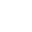• FAQ
• Contact/ Homework Answers / Physics / Question Flux and nonconducting shells. A charged particle suspended at the
Not my Question
Flag Content

# Question : Question Flux and nonconducting shells. A charged particle suspended at theQuestion

Flux and nonconducting shells. A charged particle is suspended at the center of two concentric spherical shells that are very thin and made of nonconducting material. Figure (a) shows a cross section. Figure (b) gives the net flux through a Gaussian sphere centered on the particle, as a function of the radius r of the sphere. The scale of the vertical axis is set by s = 28.0 Ý 105 N·m2/C. (a) What is the charge of the central particle? Give your answers in µC. What are the net charges of (b) shell A and (c) shell B?

Flux and nonconducting shells. A charged particle is suspended at the center of two concentric spherical shells that are very thin and made of nonconducting material. Figure (a) shows a cross section. Figure (b) gives the net flux through a Gaussian sphere centered on the particle, as a function of the radius r of the sphere. The scale of the vertical axis is set by What is the charge of the central particle? Give your answers in What are the net charges of (b) shell A and (c) shell B?

## Solution 5 (1 Ratings )

Solved
Physics 8 Months Ago 3 Views